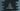# C program to print a star or X pattern using any character### C program to print a star or X pattern :

In this C programming tutorial, we will learn how to print a star or X pattern. For example, the program will print patterns like below :

``````For size 5 :
*   *
* *
*
* *
*   *

For size 7 :
*     *
*   *
* *
*
* *
*   *
*     *``````

One thing is clear from the above examples that the height and width are equal for all patterns. Let’s try to understand how to solve this problem.

### Algorithm to print the pattern :

To create one algorithm, let me first change the above pattern by replacing all blank spaces with #. It will be :

``````*#####*
#*###*#
##*#*##
###*###
##*#*##
#*###*#
*#####*``````
1. height and width are equal here, i.e. 7.
2. Think it as a 2-D matrix. A cell of the matrix will be *, if row count and column count are equal. For example, for the first row, * is printed for the [first row,first column] position, for the second row, * is printed for the [second row,second column] etc.
3. Similarly, if the column number is equal to (size - row - 1), we will print one *. For the first row, print star for 7 - 1 - 1 = 7 column, for the second row, print star for 7 - 2 - 1 = 6th column etc.
4. Print blank spaces for all other cells. Also, print one new line after each line.

That’s it. Now, we can write one program that will follow all four rules we have discussed above.

### C program :

``````#include <stdio.h>

int main()
{
//1
int i, j, size;

//2
printf("Enter size : ");
scanf("%d", &size);

//3
for (i = 0; i < size; i++)
{
//4
for (j = 0; j < size; j++)
{
//5
if (i == j || j == (size - i - 1))
{
printf("*");
}
else
{
printf(" ");
}
}
//6
printf("\n");
}
return 0;
}``````

### Explanation :

The commented numbers in the above program denote the step numbers below:

1. Create variables i,j to use in loops and size to store the size of the pattern.
2. Ask the user to enter the size. Read and save it in the size variable.
3. We are running two for loops. The outer one runs for each row and the inner one runs for each column.
4. Since, row and column count is equal, both loops will run for size amount of time.
5. Check if the current row count is equal to the current column count. Or if the current column count is (size - row-count -1). If yes, print one star. Else, print one blank space.
6. Print one new line after each row.

### Sample Output :

``````Enter size : 7
*     *
*   *
* *
*
* *
*   *
*     *

Enter size : 9
*       *
*     *
*   *
* *
*
* *
*   *
*     *
*       *

Enter size : 3
* *
*
* *

Enter size : 4
*  *
**
**
*  *
``````